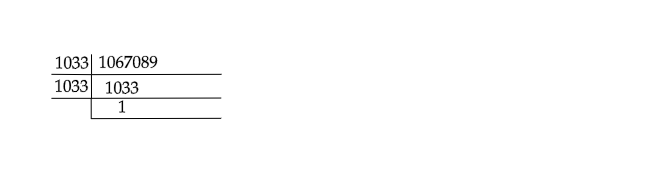# Find the square root by long division $1067089$.

Given: A number $1067089$.

To do: To find the square root of  $1067089$ by long division method.

Solution:Therefore, $\sqrt{1067089}$

$=\sqrt{1033\times1033}$

$=1033$